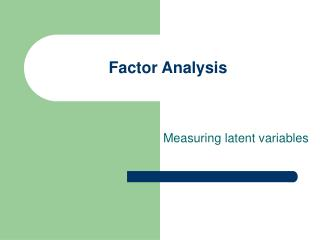# Factor Analysis - PowerPoint PPT PresentationDownload PresentationFactor Analysis

Factor Analysis
Download Presentation## Factor Analysis

- - - - - - - - - - - - - - - - - - - - - - - - - - - E N D - - - - - - - - - - - - - - - - - - - - - - - - - - -
##### Presentation Transcript

1. Factor Analysis Measuring latent variables

2. Factor Analysis - Discussion • Definition • Vocabulary • Simple Procedure • SPSS example • ICPSR and hands on

3. Definition • Factor analysis is a process by which we take a large number of variables (read that measurements) and reduce them into a smaller number of “factors”, by “extracting” the shared variation among variables. The result is a factor “loading” for each variable on each factor

4. Vocabulary and Procedure Exploratory Factor Analysis uses all of the variance of each variable to establish factors. Such technique is called “Principal Component Analysis”. It establishes the number of factors by several techniques, the two most often used are: 1) recognizing only those whose eigenvalue is > one (the Kaiser method); or 2) only those whose plot shows a vertical line (Scree method)

5. Vocabulary and Procedure (cont.) • In examining the number of factors or components, we would need to “rotate” their loadings, either Orthogonally (resulting in a loading matrix), which is the correlation between variables and the factors; • Alternatively, we can rotate them Obliquely (which results in the factors being correlated with each other). This is referred to as a factor correlation matrix. Used only when we believe the factors themselves, are correlated.

6. Orthogonal Rotation +1.0 X2 X4 X1 -1.0 +1.0 X3 -1.0

7. Vocabulary and Procedure (cont.) Interpreting loadings is quite subjective, but can be looked at largely by size. Components #1 #2 .879 .296 .846 .450 .763 .509 .279 .719 .480 .678 Variable X1 Variable X2 Variable X3 Variable X4 Variable X5

8. Steps in the Analysis • Input the data • Analyze, Dimension Reduction, Factor • Choose the extraction and rotation method • Generate the Output • Interpret the results

9. Input the data

10. Generate the Procedure

11. Produce the Output

12. Produce the Output (cont.)

13. Produce the Output (cont.)